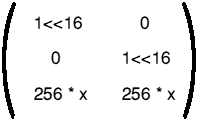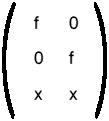# DrawFile

DrawFile is a module that renders Draw files.

##### Differences between DrawFile output and !Draw output

The following are some small differences between the output of the DrawFile module and !Draw.

###### Text

A text line that uses a font which can't be found will be rendered (in system font) at a size to fit its bounding box.

###### Transformed text

Transformed text lines in system font are supported. A transformed text line that uses a font which can't be found will be rendered (in system font) at a size to fit its bounding box. The transformation will be ignored.

###### Text areas

In a text area, if you change (for example) the margin size (\M command), the change doesn't take effect until the next output line. In Draw, this refers to printable characters: but in DrawFile, it includes colour and font change commands as well (this is because DrawFile uses the Font Manager to remember the current font and colours). This means that line breaks can happen at slightly different places when using DrawFile.

The following commands cause output to occur:

`B C U V <digits>`

The following do not:

`! ; A D F L M P`

By preceding the former with the latter, the problem can be avoided.

###### Sprite colours

For a sprite without a palette, the colours used are the WIMP colours, found by using Wimp_ReadPalette.

#### SWI DrawFile_Render

##### On entry
 R0 = flags: bit 0 set means render bounding boxes (as dotted red rectangles) bit 1 set means do not render the objects themselves bit 2 set means R5 is used as the flatness parameter R1 = pointer to Draw file data R2 = size of Draw file in bytes R3 = pointer to transformation matrix 0use identity R4 = pointer to clipping rectangle in OS units 0no clipping rectangle set up R5 = flatness with which to render lines (if bit 2 of R0 set)
##### On exit

All registers preserved

##### Use

This SWI renders a Draw file at a given screen position where that position is defined as screen position 0, 0 with the x- and y-translations as specified in the transformation matrix. Hence to render a non-rotated 1:1 Draw file at x, y (screen coordinates in OS units) the transformation matrix is:The effects of calling the module with the matrix not of the form:(which is a translation and a magnification). If R3 = 0, then unit transformation matrix is assumed (i.e. the Draw file is rendered with its bottom left corner at screen coordinates (0, 0)).

The clipping rectangle is typically a redraw rectangle returned by the Wimp on a redraw window request. If R4 = 0, then the whole Draw file is rendered. If non-zero, only objects which intersect the clipping rectangle are rendered.

##### C veneer

```extern _kernel_oserror *drawfile_render (int        flags,
void      *data,
int        size,
Transform *trfm,
BBox      *clip,
int        flatness);```

#### SWI DrawFile_BBox

##### On entry
 R0 = flags (must be 0) R1 = pointer to Draw file data R2 = size of Draw file in bytes R3 = pointer to transformation matrix 0use identity R4 = pointer to 4-word buffer to hold the bounding box of the Draw file (x0, y0, x1, y1) in Draw units
##### On exit

All registers preserved

Buffer pointed at by R4 holds the bounding box of the Draw file (x0, y0, x1, y1) in Draw units

##### Use

This SWI is used to determine the bounding box (in Draw units) of the given Draw file, as if it were plotted with the transformation given.

##### C veneer

```extern _kernel_oserror *drawfile_bbox (int        flags,
void      *data,
int        size,
Transform *trfm,
BBox      *box);```

#### SWI DrawFile_DeclareFonts

##### On entry
 R0 = flags bit 0 set means do not download font (passed to PDriver_DeclareFont) R1 = pointer to Draw file data R2 = size of Draw file in bytes
##### On exit

All registers preserved

All fonts used by the document have been declared

##### Use

If a printer requires font declarations, this SWI must be called for each Draw file to be printed, between the calls to PDriver_SelectJob and PDriver_DrawPage.

All fonts are declared as 'kerned', since this includes the non-kerned case.

##### C veneer

```extern _kernel_oserror *drawfile_declare_fonts (int   flags,
void *data,
int   size);```

This edition Copyright © 3QD Developments Ltd 2015
Last Edit: Tue,03 Nov 2015